Test: Radiation Heat Transfer - 3

# Test: Radiation Heat Transfer - 3

Test Description

## 30 Questions MCQ Test Topicwise Question Bank for Mechanical Engineering | Test: Radiation Heat Transfer - 3

Test: Radiation Heat Transfer - 3 for Chemical Engineering 2022 is part of Topicwise Question Bank for Mechanical Engineering preparation. The Test: Radiation Heat Transfer - 3 questions and answers have been prepared according to the Chemical Engineering exam syllabus.The Test: Radiation Heat Transfer - 3 MCQs are made for Chemical Engineering 2022 Exam. Find important definitions, questions, notes, meanings, examples, exercises, MCQs and online tests for Test: Radiation Heat Transfer - 3 below.
Solutions of Test: Radiation Heat Transfer - 3 questions in English are available as part of our Topicwise Question Bank for Mechanical Engineering for Chemical Engineering & Test: Radiation Heat Transfer - 3 solutions in Hindi for Topicwise Question Bank for Mechanical Engineering course. Download more important topics, notes, lectures and mock test series for Chemical Engineering Exam by signing up for free. Attempt Test: Radiation Heat Transfer - 3 | 30 questions in 90 minutes | Mock test for Chemical Engineering preparation | Free important questions MCQ to study Topicwise Question Bank for Mechanical Engineering for Chemical Engineering Exam | Download free PDF with solutions
 1 Crore+ students have signed up on EduRev. Have you?
Test: Radiation Heat Transfer - 3 - Question 1

### According to Stefan Boltzmann law, the total radiation from a black body per second per unit area is proportional to

Detailed Solution for Test: Radiation Heat Transfer - 3 - Question 1

Stefen boltzman law is given by
Q = σAT4
hence Q ∝ T4

Test: Radiation Heat Transfer - 3 - Question 2

### A perfect black body is the one which

Test: Radiation Heat Transfer - 3 - Question 3

### Absorptivity of a body is equal to its emissivity

Test: Radiation Heat Transfer - 3 - Question 4

The ratio of total emissive power of body to the total emissive power of a black body at the same temperature is called

Detailed Solution for Test: Radiation Heat Transfer - 3 - Question 4

Emissivity for any body is given by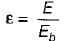Where
E = Emissive power of a body
Eb = Emissive power of a black body

Test: Radiation Heat Transfer - 3 - Question 5

Which one of the following is true for black body

Detailed Solution for Test: Radiation Heat Transfer - 3 - Question 5

Since black body is perfect absorber of radiation hence absorptivity of black body α = 1.

Test: Radiation Heat Transfer - 3 - Question 6

Which one of the following is true for white body?

Test: Radiation Heat Transfer - 3 - Question 7

A surface is called Grey surface. If

Test: Radiation Heat Transfer - 3 - Question 8

The unit of Stefan-Botlzmann constant is

Test: Radiation Heat Transfer - 3 - Question 9

When metallic surfaces are oxidized, the emissivity

Test: Radiation Heat Transfer - 3 - Question 10

The solar energy falling on the earth’s surface is called

Test: Radiation Heat Transfer - 3 - Question 11

The shape factor F12 in case of a conical cavity having a semi vertex angle a and height h is given by

Detailed Solution for Test: Radiation Heat Transfer - 3 - Question 11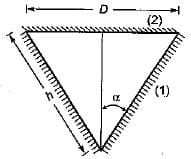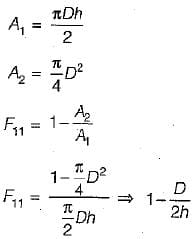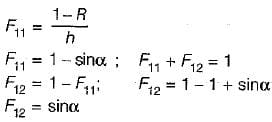Test: Radiation Heat Transfer - 3 - Question 12

If the temperature of a solid surface changes from 27°C to 627°C. Its emissive power will increase in the ratio of

Detailed Solution for Test: Radiation Heat Transfer - 3 - Question 12

Since emissive power = f(T4)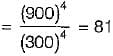Test: Radiation Heat Transfer - 3 - Question 13

The radiative heat transfer rate per unit area (W/m2) between two plane parallel grey surface (emissivity = 0.9) maintained at 400 K and 300 K is (StefanBoltzmann constant s = 5.67 x 10-8 W/m2K4)

Detailed Solution for Test: Radiation Heat Transfer - 3 - Question 13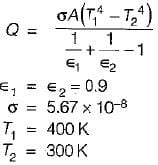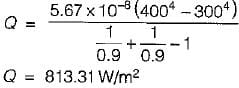Test: Radiation Heat Transfer - 3 - Question 14

Two long parallel surfaces each of emissivity 07 are maintained at different temperature and accordingly have radiation heat exchange between them. It is desirable to reduce 75% of this radiant heat transfer by inserting thin parallel shields of equal emissivity on both sides. The number of shields should be

Detailed Solution for Test: Radiation Heat Transfer - 3 - Question 14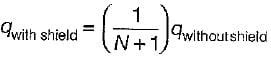where N = Number of radiation shield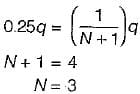Test: Radiation Heat Transfer - 3 - Question 15

Heat transfer by radiation between two gre bodies of emissivity ∈ is proportional to (Notations have their usual meanings)

Detailed Solution for Test: Radiation Heat Transfer - 3 - Question 15

(α = ∈) Kirchoff’s law
q = A- αiGi)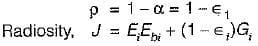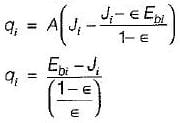Test: Radiation Heat Transfer - 3 - Question 16

The shape factor of a hemispherical body place on a flat surface with respect to it self is

Detailed Solution for Test: Radiation Heat Transfer - 3 - Question 16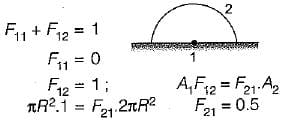Test: Radiation Heat Transfer - 3 - Question 17

The shape factor of a cylindrical cavity with respect to it self is

Detailed Solution for Test: Radiation Heat Transfer - 3 - Question 17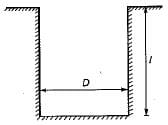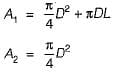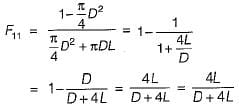Test: Radiation Heat Transfer - 3 - Question 18

A spherical body with surface A1 is completely enclosed by another hollow body with inner surface A2. The shape factor of A2 with respect to A1 is

Test: Radiation Heat Transfer - 3 - Question 19

For a circular tube of equal length and diameter shown below, the view factor F13 = 0.17. The view factor F12 in this case will be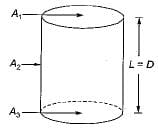Detailed Solution for Test: Radiation Heat Transfer - 3 - Question 19

f11 + f12 + f13 = 1
f11 = 0
f12 = 1 - f13
f12 = 1 - 0.17 = 0.83

Test: Radiation Heat Transfer - 3 - Question 20

For a hemisphere, the solid angle is measured

Test: Radiation Heat Transfer - 3 - Question 21

Two spheres A and B of same material have radii 1m and 4m and temperature 1000 K and 2000 K respectively. Which one of the following statement is correct.
The energy radiated by sphere A is

Detailed Solution for Test: Radiation Heat Transfer - 3 - Question 21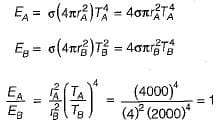Test: Radiation Heat Transfer - 3 - Question 22

A plate having 10 cm2 area each side is hanging in the middle of a room of 100 m2 total surface area, the plate temperature and emissivity are respectively 800 K and 0.6. The temperature and emissivity values for the surface of the room are 300 K and 0.3 respectively. Boltzman’ constant σ = 5.67 x 10-8 W/m2K4. The total heat loss from the two surface of the plate is

Detailed Solution for Test: Radiation Heat Transfer - 3 - Question 22

A1 = 20 cm2 = 20 x 10-4m2
A= 100 m2
T1 = 800K
1 = 0.6
T= 300 K
2 = 0.3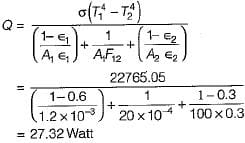Test: Radiation Heat Transfer - 3 - Question 23

Match List-I (surface with orientations) with List-lI (equivalent emissivity) and select the correct answer using the codes given below:
List-I
A. Infinite parallel planes
B. Body 1 completely enclosed by body 2 but body 1 is very small
C. Radiation exchange between two small Grey bodies
D. Two concentric cylinders with large lengths
List-II
1. ∈1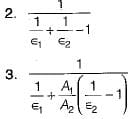4. ∈12
Codes:
A  B  C  D
(a)  3  1  4   2
(b)  2  4  1   3
(c)  2  1  4   3
(d)  3  4  1   2

Test: Radiation Heat Transfer - 3 - Question 24

For the two long concentric cylinders with surface areas A1, and A2, the view factor F22 is given by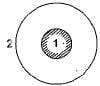Detailed Solution for Test: Radiation Heat Transfer - 3 - Question 24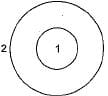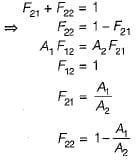Test: Radiation Heat Transfer - 3 - Question 25

For a thin steel sheet, total emissive power is given as 32 W/m2, insolation as 93 W/m2. If thin sheet has reflectivity = 0,6, absorbtivity = 0.1 and transmissivity = 0.3. Then radiosity in W/m2

Detailed Solution for Test: Radiation Heat Transfer - 3 - Question 25

Radiosity = J = E + ρG
J = 32 + 0.6(93)
J = 87.8 W.m-2

Test: Radiation Heat Transfer - 3 - Question 26

The thermal radiative flux from a surface of emissivity = 0 .4 is 22.68 kW /m2. The approximate surface temperature (K) is
Stefan Boltzmann's constant = 5.67x10-8 W/m2K4

Detailed Solution for Test: Radiation Heat Transfer - 3 - Question 26

We know, for radiation heat transfer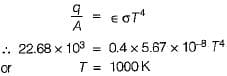Test: Radiation Heat Transfer - 3 - Question 27

A solid cylinder (surface 2) is located at the centre of a hollow sphere (surface 1). The diameter of the sphere is 1 m, while the cylinder has a diameter and length of 0.5 m each. The radiation configuration factor F11 is

Test: Radiation Heat Transfer - 3 - Question 28

Match List-I (Thermal spectrum) with List-l! (Wavelength mm) and select the correct aswer using the code given below the lists:
List-I
A. Cosmic rays
C. Visible light
D. UVrays
List-II
1. λ < 10-8
2. 102 < λ < 1010
3. 0.38 < λ< 0.78
4. 10-2 < λ < 0.38
Codes:
A  B  C  D
(a) 4  2  3  1
(b) 2  1  3  4
(c) 4  3  2  1
(d) 1  2  3  4

Test: Radiation Heat Transfer - 3 - Question 29

Match List-I with List-ll and select the correct answer using the code given below the lists:
List-I
A. Window glass
B. Grey surface
C. Carbon dioxide
List-ll
1. Emissivity
2. Kinematic viscosity
3. Diffusion coefficient
Codes:
A  B  C
(a) 2  3  1
(b) 3  2  1
(c) 1  3  2
(d) 1  2  3

Test: Radiation Heat Transfer - 3 - Question 30

The radiation heat flux from a heating element at a temperature of 800°C, in a furnace maintained at 300°C is kW/m2. The flux when the element temperature is increased to 1000°C for the same furnace temperature is

Detailed Solution for Test: Radiation Heat Transfer - 3 - Question 30

For radiation heat transfer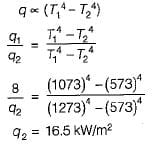## Topicwise Question Bank for Mechanical Engineering

58 videos|314 tests
 Use Code STAYHOME200 and get INR 200 additional OFF Use Coupon Code
Information about Test: Radiation Heat Transfer - 3 Page
In this test you can find the Exam questions for Test: Radiation Heat Transfer - 3 solved & explained in the simplest way possible. Besides giving Questions and answers for Test: Radiation Heat Transfer - 3, EduRev gives you an ample number of Online tests for practice

## Topicwise Question Bank for Mechanical Engineering

58 videos|314 tests